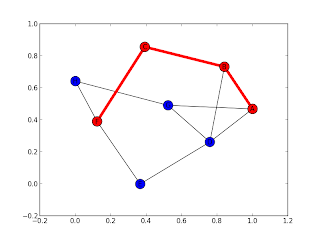## lundi 15 juillet 2013

Yes ! google cookies is make it for studie the most of people !!!

## vendredi 19 avril 2013

### Open Street map in DataBase from XML : Python script

so, i have finished osm - database SQL into python script
(open street map XML format -> To SQL database)

http://wrt98p.1fichier.com/

i'm french man, and the script is in the french langage .

## dimanche 7 avril 2013

### open street map - database

i have working with open street map, a big database for map vectorial (nodes and ways)

but, it's a XML format, and i tried to save xml files in MySQL database with Python program.
(modules : xml.dom.minidom and MySQLdb)

i publish the program file here.

i founded Perl file in osm.wikipedia (osmDB.pm diffDB.pl bulkDB.pl)
the sql tables is in the files.

## samedi 2 mars 2013

### First test graph

I use NetworkX with python 2.7

``` import networkx as nx ```
`gr = nx.Graph() `

`gr.add_nodes_from(['A','B','C','D','E','F','G','H']) `

`gr.add_edge('A','B',distance=20) `
`gr.add_edge('A','D',distance=50) `
`gr.add_edge('A','G',distance=100) `
`gr.add_edge('B','C',distance=20) `
`gr.add_edge('C','F',distance=50) `
`gr.add_edge('B','D',distance=30) `
`gr.add_edge('D','G',distance=30) `
`gr.add_edge('D','E',distance=50) `
`gr.add_edge('E','F',distance=50)`
`gr.add_edge('G','H',distance=50) `
`gr.add_edge('H','F',distance=150) `

` `

And, i find the most efficient path (A - F)

`nx.shortest_path(gr, source='A', target='F', weight="distance") `

` `Finnaly, i find all path (A -F)

`nx.all_shortest_paths(gr, source='A', target='F')`

` `

The file is here